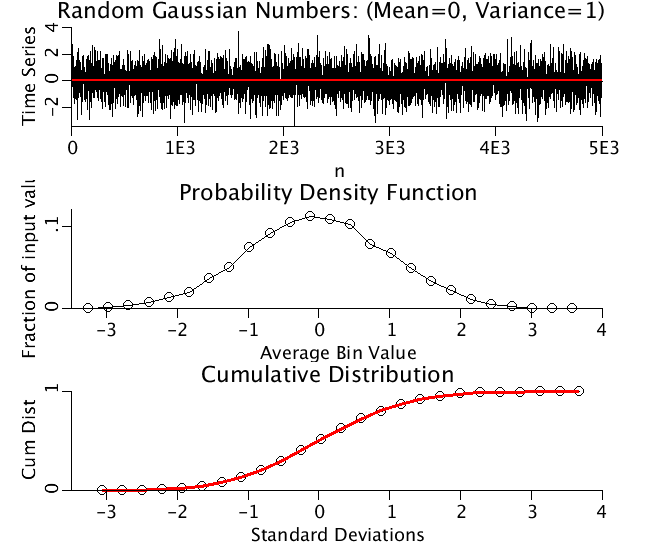# PDF

Model number
0354

Compute the PDF for a time series.

## Description

```The minimum and maximum of a time series are used to count the data
points in equally sized bins. The first bin begins at the minimum
data value and the last bin ends at the maximum data value. The
bin counts are normalized by the total number of points to produce
the probability density function. The cumulative distribution is
calculated and compared to the Gaussian distribution. The time series
used is

input(t) = Gaussian*randomg()+Uniform*random()+Constant,

where Gaussian, Uniform, and Constant are specified constands,
randomg() generates Gaussian random numbers with mean = 0 and
standard deviation = 1, and random() generates uniformly distributed
random numbers between 0 and 1.

```Upper Panel: A time series of Gaussianly distributed random numbers with mean=0, variance=4.
Middle Panel: The Probability Density Function for above time series.
Lower Panel: The Cumulative distributive function (CDF) for the above time series plotted as black circles.. CDF(x) equals the probability that the real-valued random variable X is less than or equal to x. The red line is the CDF for a Gaussian distribution.

## Equations

None.

The equations for this model may be viewed by running the JSim model applet and clicking on the Source tab at the bottom left of JSim's Run Time graphical user interface. The equations are written in JSim's Mathematical Modeling Language (MML). See the Introduction to MML and the MML Reference Manual. Additional documentation for MML can be found by using the search option at the Physiome home page.

References

None.

Key terms
Probability Density Function
pdf
time series
cumulative distribution
cum
Acknowledgements

Please cite https://www.imagwiki.nibib.nih.gov/physiome in any publication for which this software is used and send one reprint to the address given below:
The National Simulation Resource, Director J. B. Bassingthwaighte, Department of Bioengineering, University of Washington, Seattle WA 98195-5061.

Model development and archiving support at https://www.imagwiki.nibib.nih.gov/physiome provided by the following grants: NIH U01HL122199 Analyzing the Cardiac Power Grid, 09/15/2015 - 05/31/2020, NIH/NIBIB BE08407 Software Integration, JSim and SBW 6/1/09-5/31/13; NIH/NHLBI T15 HL88516-01 Modeling for Heart, Lung and Blood: From Cell to Organ, 4/1/07-3/31/11; NSF BES-0506477 Adaptive Multi-Scale Model Simulation, 8/15/05-7/31/08; NIH/NHLBI R01 HL073598 Core 3: 3D Imaging and Computer Modeling of the Respiratory Tract, 9/1/04-8/31/09; as well as prior support from NIH/NCRR P41 RR01243 Simulation Resource in Circulatory Mass Transport and Exchange, 12/1/1980-11/30/01 and NIH/NIBIB R01 EB001973 JSim: A Simulation Analysis Platform, 3/1/02-2/28/07.